# Puzzler: Six card poker22

Posted by Doug Hull,

Update: This solution was found faster than expected. I have replaced the original with a stronger solution that I was holding in reserve. Congrats to DanK for solving the original ( found here in a zip). The contest is still running with the upgraded computerHand.p

I have been known to play the occasional hand of poker, so I have this poker-inspired challenge. I made up a simple poker type of game. The deck consists of 16 cards, four each of the values 1-4. Each player is dealt six cards.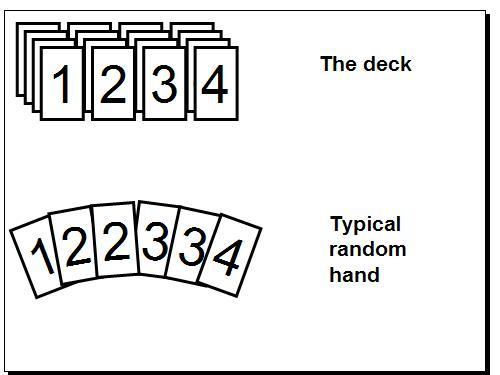Once you have the cards, you set three hands: High (3 cards), Middle (2 cards), Low (1 card). Each of these is scored differently. High hand is ranked by the sum of the cards. In the Middle hand largest pair wins. Pairs beat non-pairs, then high card wins in un-paired hands, ties broken by second card. In the Low hand, high card wins.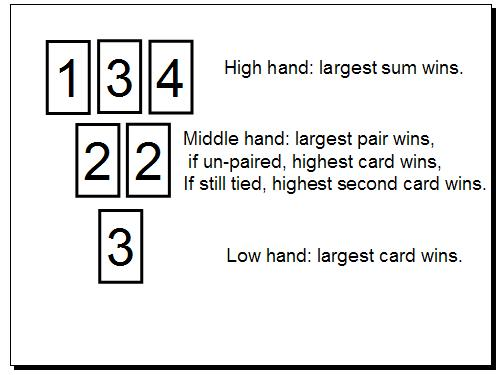Lets look at two examples with the same cards. Notice the right hand player loses or draws depending on his strategy.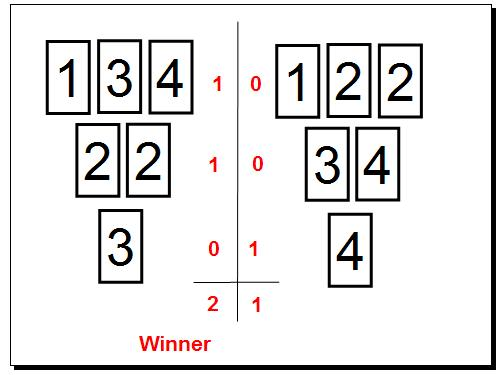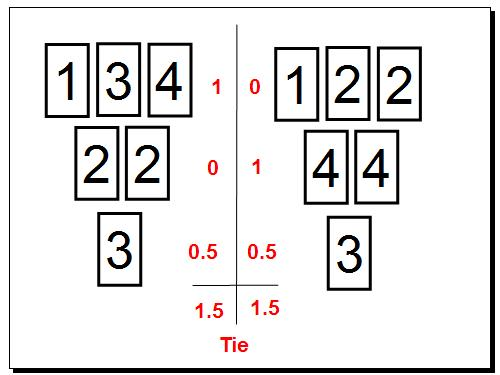You should be able to see that the right hand player could have won also!

Each hand is worth 1 point for a win, 0.5 for a draw. Win more than 1.5 of the three total points per round for the win.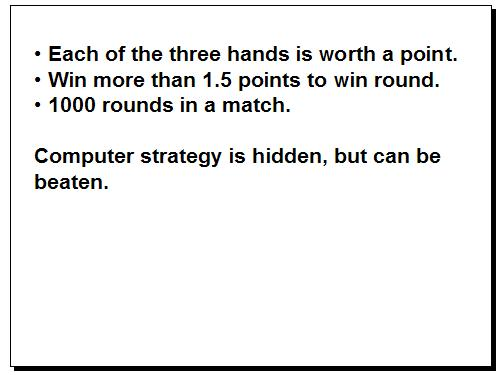I coded up a good, but simple strategy. I am confident that someone can make a strategy that will consistently win. Free MATLAB t-shirt for the first to come up with it.

Modify humanHand.m and submit it in its entirety in the comments.


function [high, middle, low] = humanHand(hand)
% Make a valid, random hand for the human
% hand will consist of six random 'cards' selected from
% [1 1 1 1 2 2 2 2 3 3 3 3 4 4 4 4];
%
% Create three hands to be judged against the computer
% doing the smae with six other cards selected from the remainder
% of the above deck.
%
% HIGH hand consists for three cards.  The value of
% the hand is the sum of the cards.  Higher is better.
%
% MIDDLE hand consists of two cards.  Any paired hand beats any non-paired hand.
% If the competeing hands are both paired, highest pair wins.  If the
% competing hands are not paired, then the highest card in each hand
% determines winner.  If highest cards are the same, second highest cards
% are compared.
%
% LOW hand consists of a single card.  Highest card wins.
%

% This is a terrible plan that makes random hands.

randomIndex = randperm(numel(hand));

hand = hand(randomIndex);

high   = hand(1:3);
middle = hand(4:5);
low    = hand(6);



Here is the code to run the contest: (No need to modify this code)


function humanPerWins = main(numPlays)

numCardsInDeck = 16;
numCardsInHand =  6;

for i = 1:numPlays;
deck = ceil(randperm(numCardsInDeck)/4);

hand.computer = sort(deck(1:2:numCardsInHand*2 - 1));
hand.human    = sort(deck(2:2:numCardsInHand*2    ));
hand.deck     = sort(deck(numCardsInHand*2 + 1:numCardsInDeck));

[highC, middleC, lowC] = computerHand(hand.computer);
[highH, middleH, lowH] =    humanHand(hand.human);

humanScore(3) = compareHigh  (  highH ,   highC);
humanScore(2) = compareMiddle(middleH , middleC);
humanScore(1) = compareLow   (   lowH ,    lowC);

humanFinal(i) = compareLow(sum(humanScore), 1.5);

end

clf
hist(humanFinal); ylim([0 numPlays])
clc
humanPerWins = sum(humanFinal)/numPlays * 100;

disp(['Your score against the computer: ' num2str(humanPerWins) '%.'])

function score = compareHigh(H, C)

if sum(H) > sum(C)
score = 1;
elseif sum(H) < sum(C)
score = 0;
else
score = 0.5;
end

function score = compareMiddle(H, C)

pairH = (H(1) == H(2));
pairC = (C(1) == C(2));

score = compareLow(pairH, pairC);

if score ~= 0.5 %is pair vs non-pair
return
else %is non-pairs or pairs

H = sort(H);
C = sort(C);

highH = H(2);
highC = C(2);

score = compareLow(highH, highC);

if score ~= 0.5
return
else
lowH = H(1);
lowC = C(1);

score = compareLow(lowH, lowC);
end
end

function score = compareLow(H, C)

if H > C
score = 1;
elseif H < C
score = 0;
else
score = 0.5;
end


Remember to use the <pre><code> and </code></pre> tags around your code in the comments.

The p-coded file that dictates the computer strategy is: computerHand.p

You can find all three files here.

Some interesting notes: My strategy against itself is about 50% win rate (of course). I tried several strategies, and used the best one overall. However, for any given "hand vs hand" one of the "lesser" strategies would beat mine. I think a blended strategy that chooses from many strategies based on the cards will win.

0 views (last 30 days)  | |